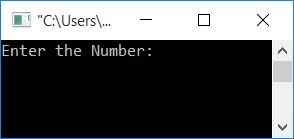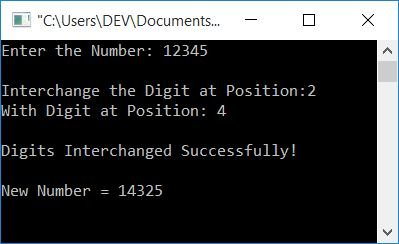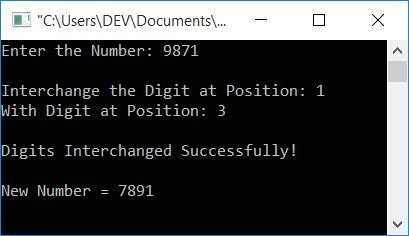# C Program to Interchange the Digits of a Number

In this article, you will learn and get code about interchanging digits of a number given by the user at run-time. Interchanging the digits of a number has been created in the following ways:

## Interchange the first and last digits of a number

Let's first create a program in C that interchanges the first and last digits of a given number.

```#include<stdio.h>
#include<conio.h>
int main()
{
int num, rem, temp, rev=0, noOfDigit=0, noOfDigitTemp, revNum, remTemp;
printf("Enter the Number: ");
scanf("%d", &num);
temp = num;
while(temp>0)
{
temp = temp/10;
noOfDigit++;
}
if(noOfDigit<2)
{
printf("\nIt's a single-digit number.");
printf("\nTo interchange the digit, enter a two or more digit number");
}
else if(noOfDigit==2)
{
temp = num;
while(temp>0)
{
rem = temp%10;
rev = (rev*10)+rem;
temp = temp/10;
}
printf("\nFirst and Last Digit Interchanged Successfully!");
printf("\n\nNew Number = %d", rev);
}
else
{
temp = num;
while(temp>0)
{
rem = temp%10;
rev = (rev*10)+rem;
temp = temp/10;
}
revNum = rev;
rev = 0;
temp = num;
noOfDigitTemp = noOfDigit;
while(temp>0)
{
remTemp = revNum%10;
if(noOfDigitTemp==noOfDigit)
{
rem = temp%10;
rev = (rev*10)+rem;
}
else if(noOfDigitTemp==1)
{
rem = temp%10;
rev = (rev*10)+rem;
}
else
{
rev = (rev*10)+remTemp;
}
temp = temp/10;
revNum = revNum/10;
noOfDigitTemp--;
}
printf("\nFirst and Last Digit Interchanged Successfully!");
printf("\n\nNew Number = %d", rev);
}
getch();
return 0;
}```

This program was built and runs under the Code::Blocks IDE. Here is its sample run:Now supply any number, say 12345, and press the ENTER key to see the following output:The following block of code:

```while(temp>0)
{
temp = temp/10;
noOfDigit++;
}```

is used to count the total number of digits available in a given number. For example, if the user enters 12345 as a number, the total number of digits is 5. If the total number of digits is less than two, the program will display a message stating that single-digit numbers cannot be interchanged. That is, to be interchangeable, it must be a two-digit or more than two-digit number. If the given number is a two-digit number, then simply reverse the number. For example, if the number is 12, after reversing, it will become 21. As you can see, its digits (first and last) are interchanged.

If there are more than 2 digits available in the given number, then program flow goes inside the else block. There, the main logic is:

• Let's suppose the user has entered 12345 as a number input.
• Reverse it and store its reverse in the rev variable that will hold 54321.
• Now we have to reverse 1 (the first digit) and 5 (the last digit) only.
• As you can see, rev holds 54321, where the first and last digits get reversed, but the rest of the three digits present in the middle also get reversed.
• Therefore, perform the reverse operation for the first and last digit on the original number, and for the rest of the digit, perform the reverse operation on the reversed number.
• That is, the original number is 12345, and the reversed number is 54321. And performing the reverse operation on the first and last digit (on the original number) with the reverse operation on the rest of the digit (on the reversed number) gives you 52341.

The dry run of the previous program with user input 12345 goes like this:

• In the beginning, num=12345, noOfDigit=5, rev=0.
• Inside the else block.
• temp=num or temp=12345.
• Using the while loop, the number gets reversed and stored in rev.
• Therefore, rev=54321.
• revNum=rev or revNum=54321, rev=0, temp=num or temp=12345, noOfDigitTemp=noOfDigit or noOfDigitTemp=5.
• Now program flow goes inside the while loop.
• The condition temp>0 or 12345>0 is true.
• Program flow goes inside the loop.
• remTemp=revNum%10 or remTemp=54321%10 or remTemp=1.
• The if block condition noOfDigitTemp==noOfDigit or 5==5 evaluates to true.
• Program flow goes inside the if block.
• rem=temp%10 or rem=12345%10 or rem=5.
• rev=(rev*10)+rem or rev=(0*10)+5 or rev=5.
• Then temp=temp/10 or temp=12345/10 or temp=1234.
• revNum=revNum/10 or revNum=54321/10 or revNum=5432.
• noOfDigitTemp-- means 1 gets subtracted from the previous value of noOfDigitTemp. Therefore noOfDigitTemp=4.
• The program flow returns to the condition of the while loop.
• That is, if temp>0 or 1234>0 evaluates to true, the program flow again goes inside the loop.
• remTemp=revNum%10 or remTemp=5432%10 or remTemp=2.
• Because noOfDigitTemp is equal to 4, and 4 is not equal to noOfDigit (5) and 1. As a result, program flow goes inside the else block.
• rev=(rev*10)+remTemp or rev=(5*10)+2 or rev=52.
• Then temp=temp/10 or temp=1234/10 or temp=123.
• revNum=revNum/10 or revNum=5432/10 or revNum=543.
• noOfDigitTemp-- means 1 gets subtracted from the previous value of noOfDigitTemp. Therefore noOfDigitTemp=3.
• The program flow now returns to the condition of the while loop.
• Continuing the same procedure, here is the updated value of each variable one by one.
• remTemp=revNum%10 or remTemp=543%10 or remTemp=3.
• Again in the else block, rev=(rev*10)+remTemp or rev=(52*10)+3 or rev=523.
• Then temp=temp/10 or temp=123/10 or temp=12.
• revNum=revNum/10 or revNum=543/10 or revNum=54.
• noOfDigitTemp=2.
• Again, remTemp=revNum%10 or remTemp=54%10 or remTemp=4.
• Again in the else block, rev=(rev*10)+remTemp or rev=(523*10)+4 or rev=5234.
• Then temp=temp/10 or temp=12/10 or temp=1.
• revNum=revNum/10 or revNum=54/10 or revNum=5.
• noOfDigitTemp=1.
• Again, remTemp=revNum%10 or remTemp=5%10 or remTemp=5.
• Because the value of noOfDigitTemp is 1. Therefore, program flow goes inside the if-else block.
• rem=temp%10 or rem=1%10 rem=1.
• rev=(rev*10)+rem or rev=(5234*10)+1 or rev=52341.
• Then temp=temp/10 or temp=1/10 or temp=0.
• revNum=revNum/10 or revNum=5/10 or revNum=0.
• noOfDigitTemp=0.
• This time the condition of the while loop, temp>0 or 0>0, evaluates to false, therefore the program flow exits the loop and prints the new number as an output.

Note: If the user enters a number that begins or ends with 0, the previous program will fail. So to overcome this problem, We have created another program using an array. That will be the complete solution to the problem of reversing a number's digits.

### Interchange the digits of a number using an array in C

The following is another program that does the same job as the previous program but uses an array. Using an array, the program becomes easier to create and understand. Just reverse the number first. And one by one, initialize all the digits in an array. Then interchange the number at the 0th index with the number at the last index.

```#include<stdio.h>
#include<conio.h>
int main()
{
int num, rem, temp, rev=0, noOfDigit=0, arr, i;
printf("Enter the Number: ");
scanf("%d", &num);
temp = num;
while(temp>0)
{
temp = temp/10;
noOfDigit++;
}
temp = num;
while(temp>0)
{
rem = temp%10;
rev = (rev*10)+rem;
temp = temp/10;
}
for(i=0; i<noOfDigit; i++)
{
rem = rev%10;
arr[i] = rem;
rev = rev/10;
}
if(noOfDigit==1)
{
printf("\nIt's a single-digit number.");
printf("\nTo interchange the digit, enter a two or more digit number");
}
else if(noOfDigit==2)
{
temp = arr;
arr = arr;
arr = temp;
printf("\nFirst and Last Digit Interchanged Successfully!");
printf("\n\nNew Number = %d%d", arr, arr);
}
else
{
i=0;
temp = arr[i];
arr[i] = arr[noOfDigit-1];
arr[noOfDigit-1] = temp;
printf("\nFirst and Last Digit Interchanged Successfully!");
printf("\n\nNew Number = ");
for(i=0; i<noOfDigit; i++)
printf("%d", arr[i]);
}
getch();
return 0;
}```

It will produce the same output as the previous program.

## Interchange the two specific digits of a given number

This program is created to interchange the two digits of a number present at any position. The number and positions entered by the user.

```#include<stdio.h>
#include<conio.h>
int main()
{
int num, posFirst, posSecond;
int rem, temp, rev=0, noOfDigit=0, arr, i;
printf("Enter the Number: ");
scanf("%d", &num);
temp = num;
while(temp>0)
{
temp = temp/10;
noOfDigit++;
}
if(noOfDigit==1)
{
printf("\nIt's a single-digit number.");
printf("\nTo interchange the digit, enter a two or more digit number");
getch();
return 0;
}
else
{
printf("\nInterchange the Digit at Position: ");
scanf("%d", &posFirst);
printf("With Digit at Position: ");
scanf("%d", &posSecond);
}
if(posFirst>noOfDigit || posSecond>noOfDigit)
printf("\nInvalid Position!");
else
{
temp = num;
while(temp>0)
{
rem = temp%10;
rev = (rev*10)+rem;
temp = temp/10;
}
for(i=0; i<noOfDigit; i++)
{
rem = rev%10;
arr[i] = rem;
rev = rev/10;
}
i=0;
temp = arr[posFirst-1];
arr[posFirst-1] = arr[posSecond-1];
arr[posSecond-1] = temp;
printf("\nDigits Interchanged Successfully!");
printf("\n\nNew Number = ");
for(i=0; i<noOfDigit; i++)
printf("%d", arr[i]);
}
getch();
return 0;
}```

Here is its sample run:Now supply any number, say 12345. Because it is a number that has more than one digit. As a result, the program prompts you to enter the digit position to be exchanged or interchanged. For example, if the user wants to swap the number in the second position with the number in the fourth position, he or she can do so. Then, simply replace the digit 2 with the digit 4, and the new number is 14325, as shown in the output:Here is another sample run, with an input number of 9871, where the digit at position first (that is 9) gets interchanged with the digit at position third (that is 7):C Quiz

« Previous Program Next Program »